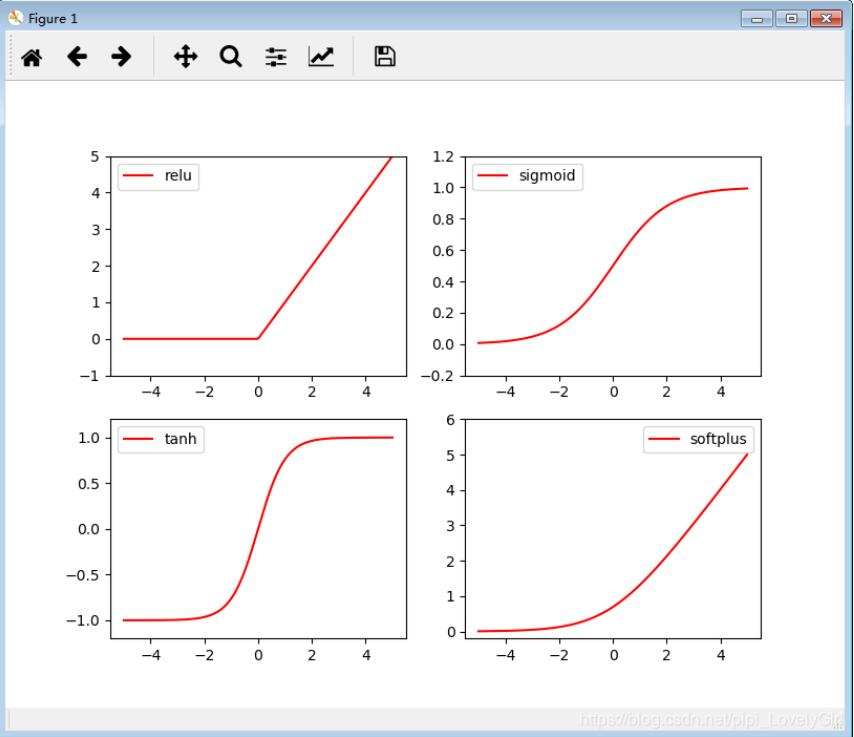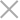# pytorch绘制曲线的方法

• 作者: 獬豸烤肉
• 来源: 51数据库
• 2021-07-12

```import torch
import torch.nn.functional as f
import matplotlib.pyplot as plt

# fake data
x = torch.linspace(-5, 5, 200) # x data (tensor), shape=(100, 1)
x = variable(x) #创建 variable（变量），构造神经网络要使用variable类型
x_np = x.data.numpy() # numpy array for plotting，用于绘图的numpy数组

# following are popular activation functions，以下是常用的激活函数
y_relu = torch.relu(x).data.numpy()
y_sigmoid = torch.sigmoid(x).data.numpy()
y_tanh = torch.tanh(x).data.numpy()
y_softplus = f.softplus(x).data.numpy() # there's no softplus in torch。torch没有softplus
# y_softmax = torch.softmax(x, dim=0).data.numpy() softmax is a special kind of activation function, it is about probability
#softmax是一种特殊的激活函数，它与概率有关

# plt to visualize these activation function
#将这些激活函数可视化
plt.figure(1, figsize=(8, 6)) # 横坐标与纵坐标
plt.subplot(221)
#plt.subplot()函数用于直接指定划分方式和位置进行绘图。
# 使用plt.subplot来创建小图. plt.subplot(221)表示将整个图像窗口分为2行2列, 当前位置为1.
plt.plot(x_np, y_relu, c='red', label='relu')
#plt.plot(x,y,format_string,**kwargs)
#x轴数据，y轴数据，format_string控制曲线的格式字串
#format_string 由颜色字符，风格字符，和标记字符
plt.ylim((-1, 5)) # 设置纵坐标的范围
plt.legend(loc='best')#plt.legend()函数的作用是给图像加图例。，就左上角relu那个
#图例是集中于地图一角或一侧的地图上各种符号和颜色所代表内容与指标的说明，有助于更好的认识地图

plt.subplot(222)# 使用plt.subplot来创建小图. plt.subplot(221)表示将整个图像窗口分为2行2列, 当前位置为2.
plt.plot(x_np, y_sigmoid, c='red', label='sigmoid')
#plt.plot(x,y,format_string,**kwargs)
#x轴数据，y轴数据，format_string控制曲线的格式字串
#format_string 由颜色字符，风格字符，和标记字符
plt.ylim((-0.2, 1.2)) # 设置纵坐标的范围
plt.legend(loc='best')#plt.legend()函数的作用是给图像加图例。，就左上角relu那个
#图例是集中于地图一角或一侧的地图上各种符号和颜色所代表内容与指标的说明，有助于更好的认识地图

plt.subplot(223)# 使用plt.subplot来创建小图. plt.subplot(221)表示将整个图像窗口分为2行2列, 当前位置为3.
plt.plot(x_np, y_tanh, c='red', label='tanh')
#plt.plot(x,y,format_string,**kwargs)
#x轴数据，y轴数据，format_string控制曲线的格式字串
#format_string 由颜色字符，风格字符，和标记字符
plt.ylim((-1.2, 1.2))# 设置纵坐标的范围
plt.legend(loc='best')#plt.legend()函数的作用是给图像加图例。，就左上角relu那个
#图例是集中于地图一角或一侧的地图上各种符号和颜色所代表内容与指标的说明，有助于更好的认识地图

plt.subplot(224)# 使用plt.subplot来创建小图. plt.subplot(221)表示将整个图像窗口分为2行2列, 当前位置为4.
plt.plot(x_np, y_softplus, c='red', label='softplus')
#plt.plot(x,y,format_string,**kwargs)
#x轴数据，y轴数据，format_string控制曲线的格式字串
#format_string 由颜色字符，风格字符，和标记字符
plt.ylim((-0.2, 6))# 设置纵坐标的范围
plt.legend(loc='best')#plt.legend()函数的作用是给图像加图例。，就左上角relu那个
#图例是集中于地图一角或一侧的地图上各种符号和颜色所代表内容与指标的说明，有助于更好的认识地图

plt.show()
#plt.show()则是将plt.imshow()处理后的函数显示出来。```Java相关